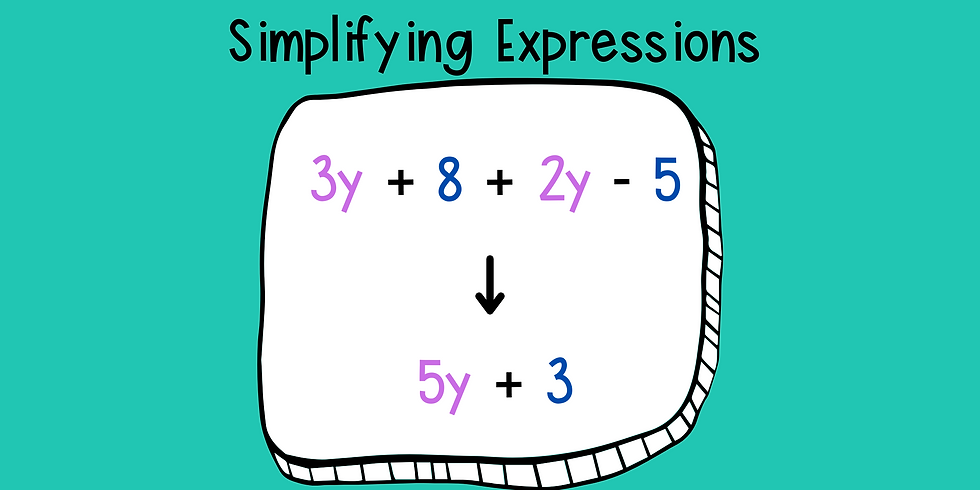top of page
Search

# Preparing for Advanced Math: Building Strong Foundations

Updated: May 7

Just here for the quiz? Alg 1, Alg 2, Geometry, Pre-Cal, Calculus

‘Spotlight on Math Anxiety’ published in 2018 by Psychology Research and Behavior Management, found that 93% of American adults “indicate that they experience some level of math anxiety”. Math anxiety has many contributing factors, including a lack of foundational skills. When students lack foundational knowledge and skills, achieving academic success gets much more difficult. This can begin in lower grade levels and travel with students to higher-level advanced math classes throughout their education.

Advanced math means any of the following courses: pre-calculus, calculus, statistics, AP calculus AB, AP calculus BC, AP statistics, college courses Math 1030 and higher, IB Math SL, HL, and Further Math.

### Before enrolling in advanced math courses, students should be able to independently...

• Complete a multiplication fact family. This will help them be able to calculate GCF and LCM quickly.

GCF (greatest common factor): This skill s needed to find factors of a number pair and use that relationship to redefine equations.

LCM (least common multiple): This skill is needed to find a common number that will multiply by both numbers within a set. This will allow students to find a common denominator in a fraction pair.

These two skills will also lead into factoring and simplifying expressions.• Factoring: Finding a common divisor within an algebraic expression. This skill is needed to simplify polynomials (expressions with multiple terms or pieces).• Advanced knowledge and understanding of mathematical vocabulary

Having a fluent math vocabulary is a vital skill because of the pace that advanced math classes move through content.• Perfect squares: Numbers that are multiples of the same number.• Square roots: Understanding the symbol √ means finding, when possible, the perfect square. Sometimes this will require factoring to pull from under the radical (the symbol of square root). These radical symbols can also have numbers outside of the symbol.• Slope and slope formula: The incline or decline of a line within a coordinate plane.• Completing the square: This math technique requires the rearrangement of terms to create perfect square from a quadratic polynomial. The idea is to take the expression you are given and pull a perfect square from it to factor the polynomial and solve.

• Basic functions and transformations: A function is the rule that defines a relationship between an independent and dependent variable. There are several types of functions that learners will need to know and understand, including but not limited to...

• linear

• exponential

• logarithmic

• absolute value

Each of these functions can be adjusted based on formulas given. These movements or adjustments are called transformations. Transformations can mean the compression, stretch, vertical/horizontal shift, or reflection of the function.

• Simplifying expressions: This means combining like terms to create a new, simpler expression.• Formula manipulation: This means rearranging parts of any given formula to be useful to the given problem. For example: If a student is given the length and area of a rectangle, they should be able to use the formula length x width= area to solve for the width.

• Using a calculator: A student's calculator is a vital tool that they can use to help them navigate their way through more rigorous math. Each calculator has special features to aid learners; they need to fully understand the tool and its capabilities to use it successfully.

### Let's talk Calculus

Calculus is often a gateway to other advanced math courses. As calculus is a higher level of math, it is necessary that the student fully understands the complexity of algebra 1 & 2, trigonometry, and geometry. These courses provide the building blocks and foundation for comprehending calculus. In addition, to these prerequisite courses, we highly suggest your student take a pre-calculus class prior to their enrollment in calculus. If pre-calculus is not offered at their school, we have a pre-calculus workshop students can attend to ensure they are ready for a calculus course.

Each quiz focuses on skills needed for various advanced math courses. Have your learner answer 5-8 questions to get a feel for how ready they are! Their score will show automatically at the end, and our math specialist will be in touch!

Looking for a detailed assessment to identify all areas of math weakness for your child? We offer a free in-depth assessment.

Math is an essential skill for success in future academic pursuits. It is the foundation for all advanced mathematics courses, such as algebra 1 and 2, geometry, and calculus. Understanding the basics of math is necessary for the comprehension of these more advanced concepts. It is also important for problem-solving and critical thinking skills, which can be helpful in a variety of everyday situations. Math is a useful tool for understanding the world around us, and it is essential for students to have a strong foundation of basic math skills in order to succeed in more advanced mathematics courses. Support throughout advanced math classes can be critical for some students. Wondering if your student needs more support? Schedule a free consultation today.